Ex 16.3

Chapter 16 Class 11 Probability (Term 2)
Serial order wise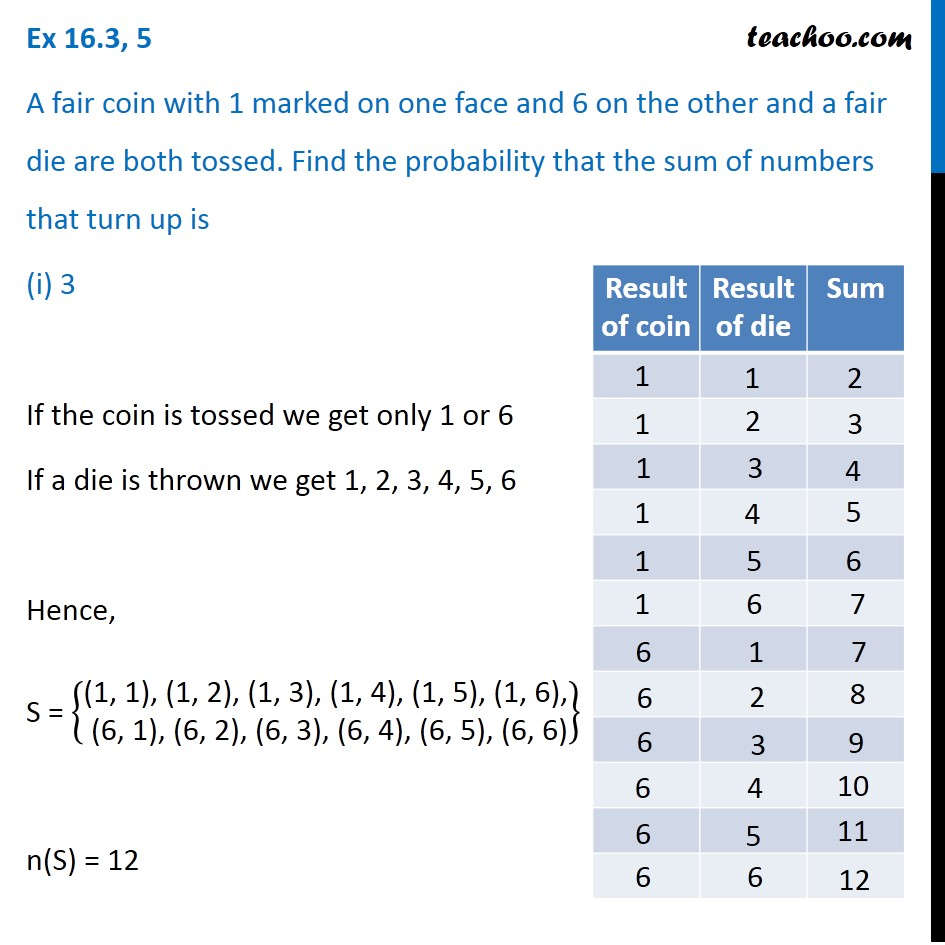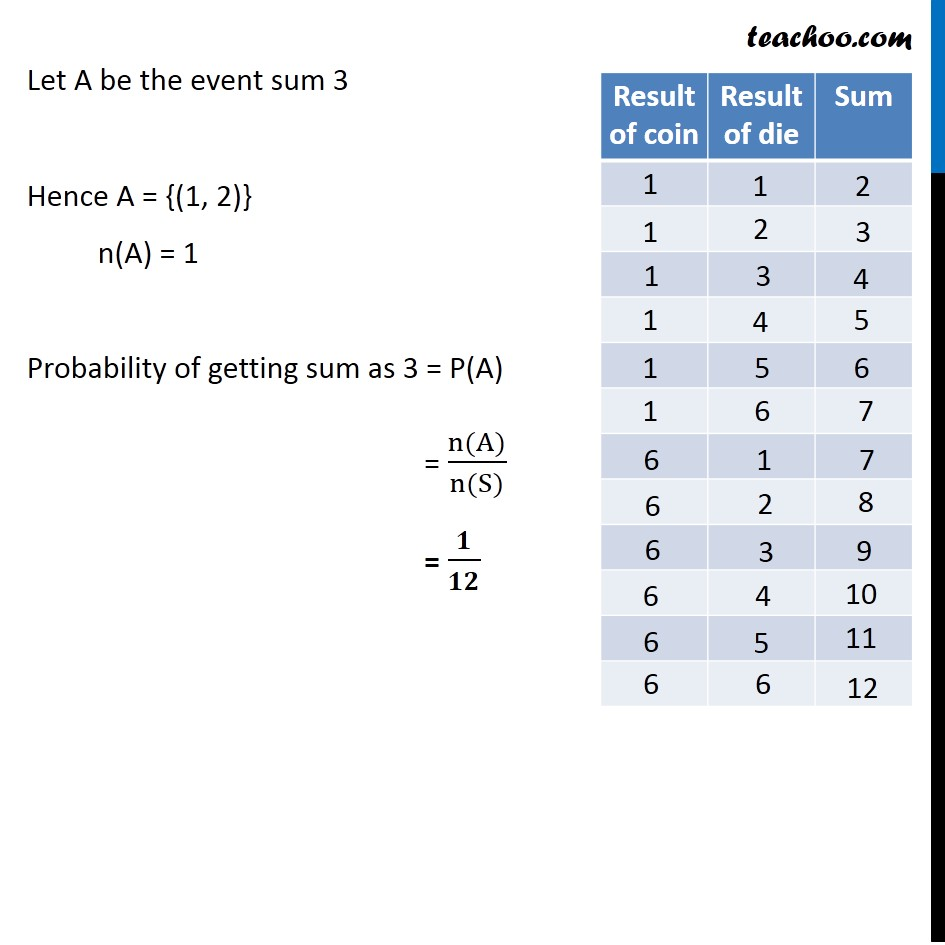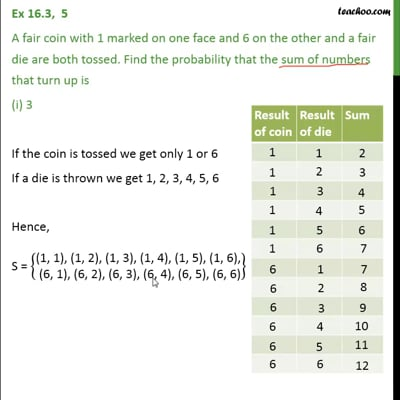This video is only available for Teachoo black users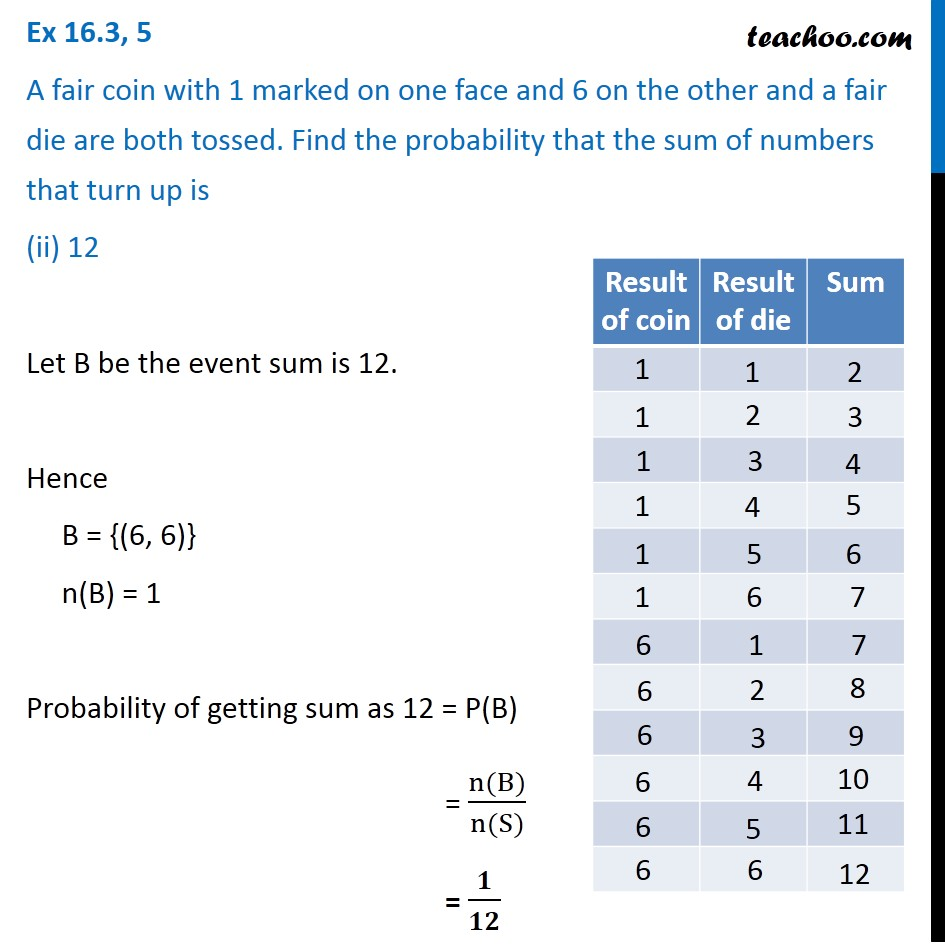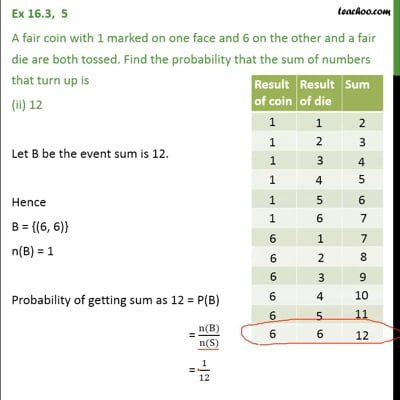This video is only available for Teachoo black users

### Transcript

Ex 16.3, 5 A fair coin with 1 marked on one face and 6 on the other and a fair die are both tossed. Find the probability that the sum of numbers that turn up is (i) 3 If the coin is tossed we get only 1 or 6 If a die is thrown we get 1, 2, 3, 4, 5, 6 Hence, S = {█("(1, 1), (1, 2), (1, 3), (1, 4), (1, 5), (1, 6)," @" (6, 1), (6, 2), (6, 3), (6, 4), (6, 5), (6, 6)" )} n(S) = 12 Let A be the event sum 3 Hence A = {(1, 2)} n(A) = 1 Probability of getting sum as 3 = P(A) = (n(A))/(n(S)) = 𝟏/𝟏𝟐 Ex 16.3, 5 A fair coin with 1 marked on one face and 6 on the other and a fair die are both tossed. Find the probability that the sum of numbers that turn up is (ii) 12 Let B be the event sum is 12. Hence B = {(6, 6)} n(B) = 1 Probability of getting sum as 12 = P(B) = (n(B))/(n(S)) = 𝟏/𝟏𝟐